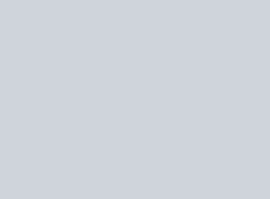From the given figure, name the following:
Question:

From the given figure, name the following:
(a) Three lines
(b) One rectilinear figure
(c) Four concurrent pointsSolution:

(a) Line $\overleftrightarrow{P Q}$, Line $\overleftrightarrow{R S}$ and Line $\overleftrightarrow{A B}$

(b) $C E F G$

(c) No point is concurrent.